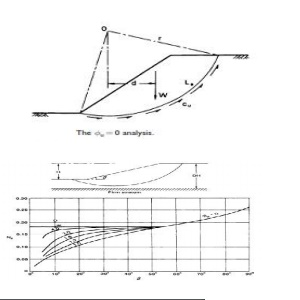Home | | Soil Mechanics | Slope Stability: Analysis For The Case Of Deta

# Slope Stability: Analysis For The Case Of DetaThis analysis, in terms of total stress, covers the case of fully saturated clay under undrained conditions, i.e. for the condition immediately after construction.

ANALYSIS FOR THE CASE OF ?  =

This analysis, in terms of total stress, covers the case of fully saturated clay under undrained conditions, i.e. for the condition immediately after construction. Only moment equilibrium is considered in the analysis. In section, the potential failure surface is assumed to be a circular arc. A trial failure surface (centre O, radius r and length La) is shown in Figure. Potential instability is due to the total weight of the soil mass (W per unit length) above the failure surface. For equilibrium the shear strength which must be mobilized along the failure surface is expressed as n=cu/Fwhere F is the factor of safety with respect to shear strength. Equating moments about O

Wd=Cu/F . LV

The moments of any additional forces must be taken into account. In the event of a tension crack developing, the arc length La is shortened and a hydrostatic force will act normal to the crack if it fills with water. It is necessary to analyze the slope for a number of trial failure surfaces in order that the minimum factor of safety can be determined.

Based on the principle of geometric similarity, Taylor  published stability coefficients for the analysis of homogeneous slopes in terms of total stress. For a slope of height H the stability coefficient (Ns) for the failure surface along which the factor of safety is a

Tm=Pi/F . Cu/FStudy Material, Lecturing Notes, Assignment, Reference, Wiki description explanation, brief detail

Related Topics